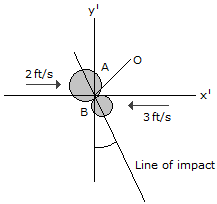# Engineering Mechanics - KOP: Impulse and Momentum - Discussion

### Discussion :: KOP: Impulse and Momentum - General Questions (Q.No.5)

5.Two coins A and B have the initial velocities shown just before they collide at point O. If they have weights of WA = 13.2(10-3) lb and WB = 6.6(10-3) lb and the surface upon which they slide is smooth, determine their speed just after impact. The coefficient of restitution is e = 0.65.

 [A]. vA2 = 1.856 ft/s, vB2 = 3.18 ft/s [B]. vA2 = 1.871 ft/s, vB2 = 2.76 ft/s [C]. vA2 = 1.772 ft/s, vB2 = 2.88 ft/s [D]. vA2 = 1.755 ft/s, vB2 = 2.92 ft/s

Explanation:

No answer description available for this question.